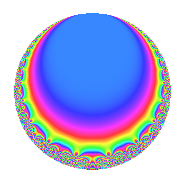# Properties

 Label 20.4.c.aLevel 20 Weight 4 Character orbit 20.c Analytic conductor 1.180 Analytic rank 0 Dimension 2 CM No Inner twists 2

# Related objects

## Newspace parameters

 Level: $$N$$ = $$20 = 2^{2} \cdot 5$$ Weight: $$k$$ = $$4$$ Character orbit: $$[\chi]$$ = 20.c (of order $$2$$ and degree $$1$$)

## Newform invariants

 Self dual: No Analytic conductor: $$1.18003820011$$ Analytic rank: $$0$$ Dimension: $$2$$ Coefficient field: $$\Q(\sqrt{-19})$$ Coefficient ring: $$\Z[a_1, a_2, a_3]$$ Coefficient ring index: $$2^{2}$$ Sato-Tate group: $\mathrm{SU}(2)[C_{2}]$

## $q$-expansion

Coefficients of the $$q$$-expansion are expressed in terms of $$\beta = 2\sqrt{-19}$$. We also show the integral $$q$$-expansion of the trace form.

 $$f(q)$$ $$=$$ $$q$$ $$-\beta q^{3}$$ $$+ ( 7 + \beta ) q^{5}$$ $$+ \beta q^{7}$$ $$-49 q^{9}$$ $$+O(q^{10})$$ $$q$$ $$-\beta q^{3}$$ $$+ ( 7 + \beta ) q^{5}$$ $$+ \beta q^{7}$$ $$-49 q^{9}$$ $$+ 20 q^{11}$$ $$+ 6 \beta q^{13}$$ $$+ ( 76 - 7 \beta ) q^{15}$$ $$-8 \beta q^{17}$$ $$-84 q^{19}$$ $$+ 76 q^{21}$$ $$-7 \beta q^{23}$$ $$+ ( -27 + 14 \beta ) q^{25}$$ $$+ 22 \beta q^{27}$$ $$+ 6 q^{29}$$ $$-224 q^{31}$$ $$-20 \beta q^{33}$$ $$+ ( -76 + 7 \beta ) q^{35}$$ $$-14 \beta q^{37}$$ $$+ 456 q^{39}$$ $$+ 266 q^{41}$$ $$+ 35 \beta q^{43}$$ $$+ ( -343 - 49 \beta ) q^{45}$$ $$-43 \beta q^{47}$$ $$+ 267 q^{49}$$ $$-608 q^{51}$$ $$+ 42 \beta q^{53}$$ $$+ ( 140 + 20 \beta ) q^{55}$$ $$+ 84 \beta q^{57}$$ $$-28 q^{59}$$ $$+ 182 q^{61}$$ $$-49 \beta q^{63}$$ $$+ ( -456 + 42 \beta ) q^{65}$$ $$-49 \beta q^{67}$$ $$-532 q^{69}$$ $$+ 408 q^{71}$$ $$-124 \beta q^{73}$$ $$+ ( 1064 + 27 \beta ) q^{75}$$ $$+ 20 \beta q^{77}$$ $$+ 48 q^{79}$$ $$+ 349 q^{81}$$ $$+ 23 \beta q^{83}$$ $$+ ( 608 - 56 \beta ) q^{85}$$ $$-6 \beta q^{87}$$ $$-1526 q^{89}$$ $$-456 q^{91}$$ $$+ 224 \beta q^{93}$$ $$+ ( -588 - 84 \beta ) q^{95}$$ $$-64 \beta q^{97}$$ $$-980 q^{99}$$ $$+O(q^{100})$$ $$\operatorname{Tr}(f)(q)$$ $$=$$ $$2q$$ $$\mathstrut +\mathstrut 14q^{5}$$ $$\mathstrut -\mathstrut 98q^{9}$$ $$\mathstrut +\mathstrut O(q^{10})$$ $$2q$$ $$\mathstrut +\mathstrut 14q^{5}$$ $$\mathstrut -\mathstrut 98q^{9}$$ $$\mathstrut +\mathstrut 40q^{11}$$ $$\mathstrut +\mathstrut 152q^{15}$$ $$\mathstrut -\mathstrut 168q^{19}$$ $$\mathstrut +\mathstrut 152q^{21}$$ $$\mathstrut -\mathstrut 54q^{25}$$ $$\mathstrut +\mathstrut 12q^{29}$$ $$\mathstrut -\mathstrut 448q^{31}$$ $$\mathstrut -\mathstrut 152q^{35}$$ $$\mathstrut +\mathstrut 912q^{39}$$ $$\mathstrut +\mathstrut 532q^{41}$$ $$\mathstrut -\mathstrut 686q^{45}$$ $$\mathstrut +\mathstrut 534q^{49}$$ $$\mathstrut -\mathstrut 1216q^{51}$$ $$\mathstrut +\mathstrut 280q^{55}$$ $$\mathstrut -\mathstrut 56q^{59}$$ $$\mathstrut +\mathstrut 364q^{61}$$ $$\mathstrut -\mathstrut 912q^{65}$$ $$\mathstrut -\mathstrut 1064q^{69}$$ $$\mathstrut +\mathstrut 816q^{71}$$ $$\mathstrut +\mathstrut 2128q^{75}$$ $$\mathstrut +\mathstrut 96q^{79}$$ $$\mathstrut +\mathstrut 698q^{81}$$ $$\mathstrut +\mathstrut 1216q^{85}$$ $$\mathstrut -\mathstrut 3052q^{89}$$ $$\mathstrut -\mathstrut 912q^{91}$$ $$\mathstrut -\mathstrut 1176q^{95}$$ $$\mathstrut -\mathstrut 1960q^{99}$$ $$\mathstrut +\mathstrut O(q^{100})$$

## Character Values

We give the values of $$\chi$$ on generators for $$\left(\mathbb{Z}/20\mathbb{Z}\right)^\times$$.

 $$n$$ $$11$$ $$17$$ $$\chi(n)$$ $$1$$ $$-1$$

## Embeddings

For each embedding $$\iota_m$$ of the coefficient field, the values $$\iota_m(a_n)$$ are shown below.

For more information on an embedded modular form you can click on its label.

Label $$\iota_m(\nu)$$ $$a_{2}$$ $$a_{3}$$ $$a_{4}$$ $$a_{5}$$ $$a_{6}$$ $$a_{7}$$ $$a_{8}$$ $$a_{9}$$ $$a_{10}$$
9.1
 0.5 + 2.17945i 0.5 − 2.17945i
0 8.71780i 0 7.00000 + 8.71780i 0 8.71780i 0 −49.0000 0
9.2 0 8.71780i 0 7.00000 8.71780i 0 8.71780i 0 −49.0000 0
 $$n$$: e.g. 2-40 or 990-1000 Significant digits: Format: Complex embeddings Normalized embeddings Satake parameters Satake angles

## Inner twists

Char. orbit Parity Mult. Self Twist Proved
1.a Even 1 trivial yes
5.b Even 1 yes

## Hecke kernels

There are no other newforms in $$S_{4}^{\mathrm{new}}(20, [\chi])$$.